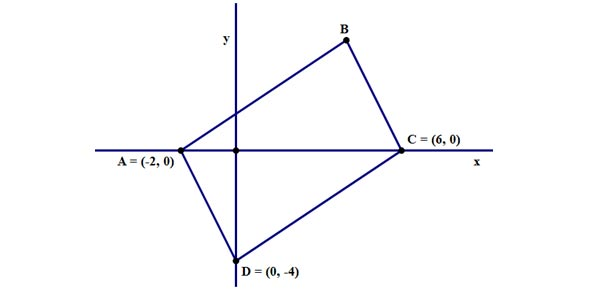# Coordinate Geometry: Mid-points

5 Questions | Total Attempts: 245SettingsNumber exactly between 4 and 12 is 8 = (4+12)/2Number exactly between - 4 and 12 = ( - 4+12)/2 = 4Given two points (x1,y1) & (x2,y2),Midpoint = ( (x1+x2)/2, (y1+y2)/2 )

Related Topics
• 1.
Find the midpoint between (3,5) and (9,1)
• A.

(12,6)

• B.

(6,6)

• C.

(6,3)

• D.

(3,2)

• 2.
(4,5) is the midpoint between (1,2) and D.Find the coordinates of D.
• A.

(3,8)

• B.

(7,8)

• C.

(3,3)

• D.

(5,7)

• 3.
Find the midpoint between ( -1, 5) and (-11, 11)
• A.

( -12, 16)

• B.

(10,6)

• C.

( -6, 8)

• D.

(6, 3)

• 4.
Given A(2,5), B(5,8), C(4,-3) and D forms a parallelogram ABCD.Find the coordinates of D.
• A.

(1, 6)

• B.

(1, - 6)

• C.

(3, 1)

• D.

(1, 3)

• 5.
Find the value of k given (4,5) is the midpoint between (-2, 3) and (k,k-3).
• A.

1

• B.

4

• C.

7

• D.

10